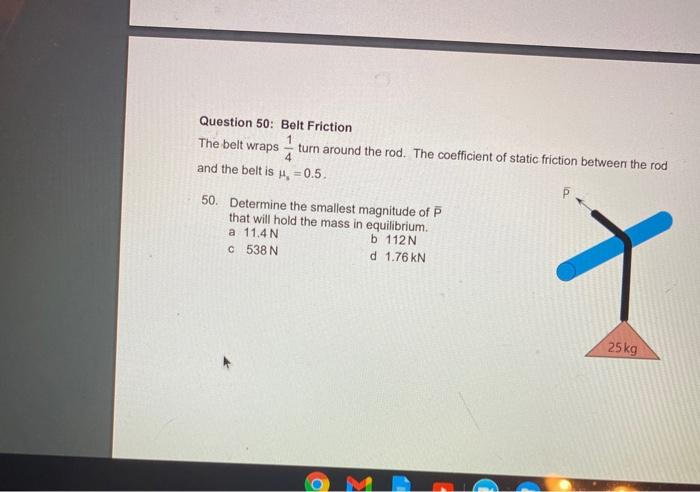# Question Question 50: Belt Friction 1 The belt wraps turn around the rod. The coefficient of static friction between the rod 4 and the belt is , =0.5. 50. Determine the smallest magnitude of P that will hold the mass in equilibrium. a 11.4 N b 112N 538N d 1.76 KN X 25 kgQYDFQI The Asker · Civil EngineeringTranscribed Image Text: Question 50: Belt Friction 1 The belt wraps turn around the rod. The coefficient of static friction between the rod 4 and the belt is , =0.5. 50. Determine the smallest magnitude of P that will hold the mass in equilibrium. a 11.4 N b 112N 538N d 1.76 KN X 25 kg
More
Transcribed Image Text: Question 50: Belt Friction 1 The belt wraps turn around the rod. The coefficient of static friction between the rod 4 and the belt is , =0.5. 50. Determine the smallest magnitude of P that will hold the mass in equilibrium. a 11.4 N b 112N 538N d 1.76 KN X 25 kg